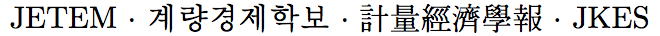Journal of Economic Theory and Econometrics: Journal of the Korean Econometric Society
Home

Archive

Search

Journal of Economic Theory and EconometricsJournal of the Korean Econometric Society

• #### Hosin Song   (Ewha Womans University)Abstract

In this paper, I propose a simulated integrated conditional moment (SICM) estimator for the binary choice model. The asymptotic property of the proposed SICM estimator is explored via Monte Carlo experiment since its asymptotic theory has not been fully developed. In particular, the SICM estimator is compared with method of simulated moment (MSM) and ML estimators by adopting a simple parametric distributional setup in the experiment. The experiment results show that the proposed SICM estimator is valid in the sense that it is consistent and its Monte Carlo variance decreases by 1=n times as the sample size increases. In particular, it is found that the variance of the SICM estimator is approximately twice that of the MSM estimator with one simulator.

Keywords
simulated integrated conditional moment, method of simulated moment, binary choice model

JEL classification codes
C15, C18, C25